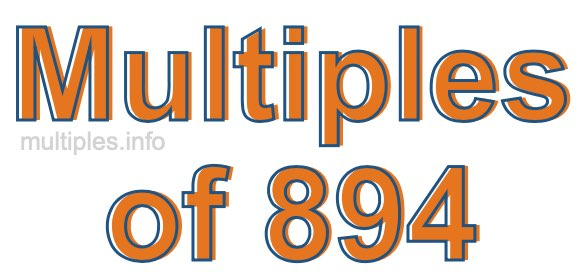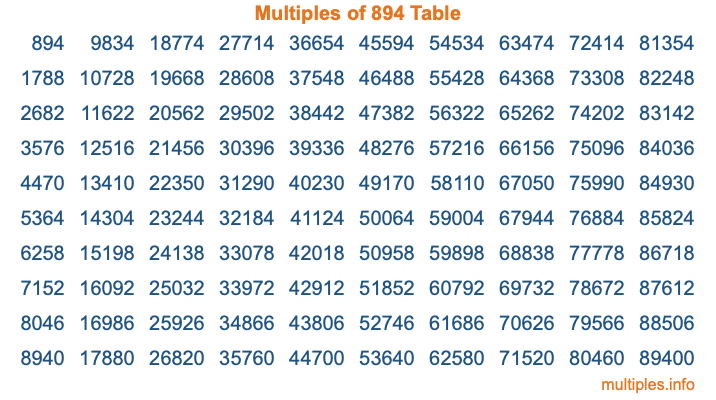Multiples of 894Welcome to the Multiples of 894 page. Here we will first teach you everything you will ever need to know about the multiples of 894, and then give you a study guide summary of everything we taught you to make sure you remember it all. Use this page to look up facts and learn information about the multiples of 894. This page will make you a multiples of eight hundred ninety-four expert!

Definition of Multiples of 894
Multiples of 894 are all the numbers that when divided by 894 equal an integer. Each of the multiples of 894 are called a multiple. A multiple of 894 is created by multiplying 894 by an integer.

Therefore, to create a list of multiples of 894, you start with 1 multiplied by 894, then 2 multiplied by 894, then 3 multiplied by 894, and so on for as long as you want. Thus, the list of the first five multiples of 894 is 894, 1788, 2682, 3576, and 4470. To see a larger list of multiples of 894, see the printable image of Multiples of 894 further down on this page. We also have a category where you can choose any nth multiple of 894.

Multiples of 894 Checker
The Multiples of 894 Checker below checks to see if any number of your choice is a multiple of 894. In other words, it checks to see if there is any number (integer) that when multiplied by 894 will equal your number. To do that, we divide your number by 894. If the the quotient is an integer, then your number is a multiple of 894.

Is  a multiple of 894?

Least Common Multiple of 894 and ...
A Least Common Multiple (LCM) is the lowest multiple that two or more numbers have in common. This is also called the smallest common multiple or lowest common multiple and is useful to know when you are adding our subtracting fractions. Enter one or more numbers below (894 is already entered) to find the LCM.

Check out our LCM Calculator if you need more details about the Least Common Multiple or if you need the LCM for different numbers for adding and subtraction fractions.

nth Multiple of 894
As we stated above, 894 is the first multiple of 894, 1788 is the second multiple of 894, 2682 is the third multiple of 894, and so on. Enter a number below to find the nth multiple of 894.

th multiple of 894

Multiples of 894 vs Factors of 894
894 is a multiple of 894 and a factor of 894, but that is where the similarities end. All postive multiples of 894 are 894 or greater than 894. All positive factors of 894 are 894 or less than 894.

Below is the beginning list of multiples of 894 and the factors of 894 so you can compare:

Multiples of 894: 894, 1788, 2682, 3576, 4470, etc.

Factors of 894: 1, 2, 3, 6, 149, 298, 447, 894

As you can see, the multiples of 894 are all the numbers that you can divide by 894 to get a whole number. The factors of 894, on the other hand, are all the whole numbers that you can multiply by another whole number to get 894.

It's also interesting to note that if a number (x) is a factor of 894, then 894 will also be a multiple of that number (x).

Multiples of 894 vs Divisors of 894
The divisors of 894 are all the integers that 894 can be divided by evenly. Below is a list of the divisors of 894.

Divisors of 894: 1, 2, 3, 6, 149, 298, 447, 894

The interesting thing to note here is that if you take any multiple of 894 and divide it by a divisor of 894, you will see that the quotient is an integer.

Multiples of 894 Table
Below is an image of the first 100 multiples of 894 in a table. The table is in chronological order, column by column. The first column has the first ten multiples of 894, the second column has the next ten multiples of 894, and so on.The Multiples of 894 Table is also referred to as the 894 Times Table or Times Table of 894. You are welcome to print out our table for your studies.

Negative Multiples of 894
Although not often discussed or needed in math, it is worth mentioning that you can make a list of negative multiples of 894 by multiplying 894 by -1, then by -2, then by -3, and so on, to get the following list of negative multiples of 894:

-894, -1788, -2682, -3576, -4470, etc.

Multiples of 894 Summary
Below is a summary of important Multiples of 894 facts that we have discussed on this page. To retain the knowledge on this page, we recommend that you read through the summary and explain to yourself or a study partner why they hold true.

There are an infinite number of multiples of 894.

A multiple of 894 divided by 894 will equal a whole number.

894 divided by a factor of 894 equals a divisor of 894.

The nth multiple of 894 is n times 894.

The largest factor of 894 is equal to the first positive multiple of 894.

894 is a multiple of every factor of 894.

894 is a multiple of 894.

A multiple of 894 divided by a divisor of 894 equals an integer.

894 divided by a divisor of 894 equals a factor of 894.

Any integer times 894 will equal a multiple of 894.

Multiples of a Number
Here you can get the multiples of another number, all with the same attention to detail as we did for multiples of 894 on this page.

Multiples of
Multiples of 895
Did you find our page about multiples of eight hundred ninety-four educational? Do you want more knowledge? Check out the multiples of the next number on our list!